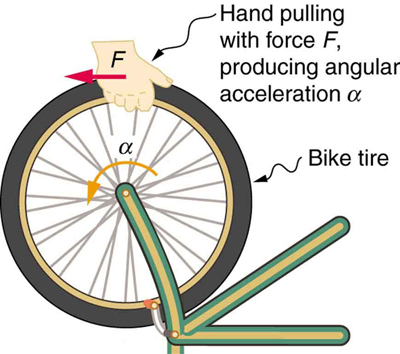Skip to main content
$$\require{cancel}$$

# 10.6: Dynamics

learning objectives

• Explain the relationship between the force, mass, radius, and angular acceleration

If you have ever spun a bike wheel or pushed a merry-go-round, you have experienced the force needed to change angular velocity. Our intuition is reliable in predicting many of the factors that are involved. For example, we know that a door opens slowly if we push too close to its hinges. Furthermore, we know that the more massive the door, the more slowly it opens. The first example implies that the farther the force is applied from the pivot, the greater the angular acceleration; another implication is that angular acceleration is inversely proportional to mass. These relationships should seem very similar to the familiar relationships among force, mass, and acceleration embodied in Newton’s second law of motion. There are, in fact, precise rotational analogs to both force and mass.

Rotational inertia, as illustrated in, is the resistance of objects to changes in their rotation. In other words, a rotating object will stay rotating and a non-rotating object will stay non-rotating unless acted on by a torque. This should remind you of Newton’s First Law.Rotational Inertia: Force is required to spin the bike wheel. The greater the force, the greater the angular acceleration produced. The more massive the wheel, the smaller the angular acceleration. If you push on a spoke closer to the axle, the angular acceleration will be smaller.

To develop the precise relationship among force, mass, radius, and angular acceleration, consider what happens if we exert a force F on a point mass m that is at a distance r from a pivot point. Because the force is perpendicular to r, an acceleration $$\mathrm{a=\frac{F}{m}}$$ is obtained in the direction of F. We can rearrange this equation such that F=ma and then look for ways to relate this expression to expressions for rotational quantities. We note that a=rα, and we substitute this expression into F=ma, yielding:

$\mathrm{F=mrα.}$

Recall that torque is the turning effectiveness of a force. In this case, because F is perpendicular to r, torque is simply $$\mathrm{τ=Fr}$$. So, if we multiply both sides of the equation above by r, we get torque on the left-hand side. That is, $\mathrm{rF = mr^2α}$, or

$\mathrm{τ = mr^2α.}$

This equation is the rotational analog of Newton’s second law ($$\mathrm{F=ma}$$), where torque is analogous to force, angular acceleration is analogous to translational acceleration, and mr2 is analogous to mass (or inertia). The quantity mr2 is called the rotational inertia or moment of inertia of a point mass m a distance r from the center of rotation.

Different shapes of objects have different rotational inertia which depend on the distribution of their mass.

## Key Points

• The farther the force is applied from the pivot, the greater the angular acceleration.
• Angular acceleration is inversely proportional to mass.
• The equation $$\mathrm{τ = mr^2α.}$$ is the rotational analog of Newton’s second law ($$\mathrm{F=ma}$$), where torque is analogous to force, angular acceleration is analogous to translational acceleration, and $$\mathrm{mr^2}$$ is analogous to mass (or inertia ).

## Key Terms

• rotational inertia: The tendency of a rotating object to remain rotating unless a torque is applied to it.
• torque: A rotational or twisting effect of a force; (SI unit newton-meter or Nm; imperial unit foot-pound or ft-lb)

LICENSES AND ATTRIBUTIONS

CC LICENSED CONTENT, SHARED PREVIOUSLY

CC LICENSED CONTENT, SPECIFIC ATTRIBUTION

• Was this article helpful?